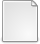# Algorithmic aspects of fixed-size coalition formation

McKay, Michael (2022) Algorithmic aspects of fixed-size coalition formation. PhD thesis, University of Glasgow.

Full text available as:PDF Download (1MB)

## Abstract

We study algorithmic aspects of models in which a set of agents is to be organised into coalitions of a fixed size. Such models can be viewed as a type of hedonic game, coalition formation game, or multidimensional matching problem. We mostly consider models in which coalitions have size three and are formalisms of Three-Dimensional Roommates (3DR). Models of 3DR are characterised by a combination of the system by which agents have preferences over coalitions, and the solution concept (e.g. stability). Since the computational problems associated with 3DR are typically hard, we consider approximate solutions and restricted cases, with the aim of characterising the boundary between tractable and intractable variants.

Part of our contribution relates to two new models of 3DR, which involve two existing systems of preferences called [B- and W-preferences]. In each model, we consider the existence of matchings that are stable. We show that the related decision problems are NP-complete and devise approximation algorithms for corresponding optimisation problems.

In a model of 3DR with additively separable preferences, we investigate stable matchings and envy-free matchings, for three successively weaker definitions of envy-freeness. We consider restrictions on the agents’ preferences including symmetric, binary, and ternary valuations. We identify dichotomies based on these restrictions and provide a comprehensive complexity classification. Interestingly, we identify a general trend that, for successively weaker solution concepts, existence and polynomial-time solvability hold under successively weaker preference restrictions.

We also consider a variant of 3DR known as Three-Dimensional Stable Matching with Cyclic preferences (3-DSM-CYC), which has been of independent interest. It was recently shown that finding a stable matching is NP-hard, so we consider a related optimisation problem and present an approximation algorithm based on serial dictatorship. We also consider a situation in which the preferences of some agents are sufficiently similar to some master list, and show that the approximation ratio of this algorithm can be improved in relation to a specific similarity measure.

Finally, we consider a problem in graph theory that generalises the notion of assigning agents to coalitions of a fixed size. Rather than organising a set of agents, the problem is to find a maximum-cardinality set of r-cliques in an undirected graph subject to that set being either vertex disjoint or edge disjoint, for a fixed integer r ≥ 3. This general problem is known as the Kr- packing problem. Here we study the restriction of this problem in which the graph has a fixed maximum degree ∆. It is known for r = 3 that the vertex-disjoint (edge-disjoint) variant is solvable in linear time if ∆ = 3 (∆ = 4) but APX-hard if ∆ ≥ 4 (∆ ≥ 5). We generalise these results to an arbitrary but fixed r ≥ 3, and provide a full complexity classification for both the vertex- and edge-disjoint variants in graphs of maximum degree ∆, for all r ≥ 3.

Item Type: Thesis (PhD) Doctoral Q Science > QA Mathematics > QA75 Electronic computers. Computer science College of Science and Engineering > School of Computing Science Manlove, Professor David 2022 Theses Team glathesis:2022-83367 Copyright of this thesis is held by the author. 19 Jan 2023 09:39 19 Jan 2023 14:38 10.5525/gla.thesis.83367 http://theses.gla.ac.uk/id/eprint/83367 Article DOIView Item